# 坐标系、点、线、面 —— 计算机几何 #

## 二维（Two Dimensional） #

### 直线（Lines） #

xy 平面上的直线可以用以下方程式表示:

``````Ax + By + C = 0
``````

`B` 不等于 0 时，方程可以转化为如下等式，`k =-A/B`, `b=-C/B`

``````y = kx + b
``````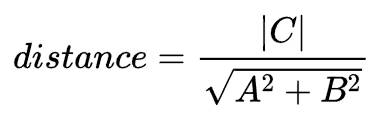### 平行直线与垂直直线 #

``````Ax + By + C = 0
Ex + Fy + G = 0
``````

``````-A/B = -E/F
``````

``````-A/B = -E/F
-C/B = -G/F
``````

``````(A/B) * (E/F) = -1
``````

## 三维（Three Dimensional） #

### 平面（Plane） #

``````Ax + By + Cz + D = 0
``````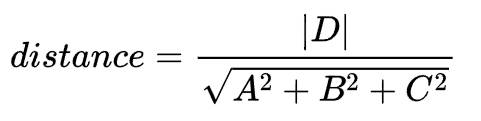## 向量（Vectors） #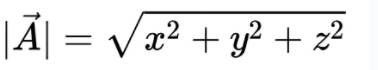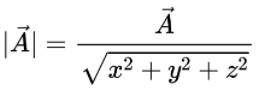### 点积（Inner Product，Dot Product） #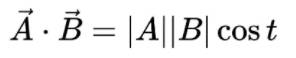1. 点积为 0，且两个向量 AB 均为非 0 向量，则两个向量垂直。
2. 点积为 1，且两个向量 AB 均为非 0 向量，则两个向量平行，且方向相同。
3. 点积为 -1，且两个向量 AB 均为非 0 向量，则两个向量平行，且方向相反。

### 直线和线段的向量表示 #

``````startPoint + t * direction
``````

`startPoint` 为线段的起始点，`direction` 为线段方向的单位向量，t 为线段的长度，所以线段的终点为 `startPoint + t * direction`

### 平面的向量表示 #

``````(P - B)n = 0
``````

### 叉积（Cross Product） #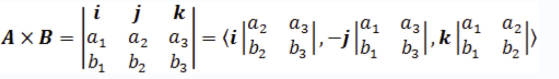``````|A|*|B|*sin(t)
``````

t 为两个向量的夹角。所以我们也可以用它求解三角形的面积。

（完）